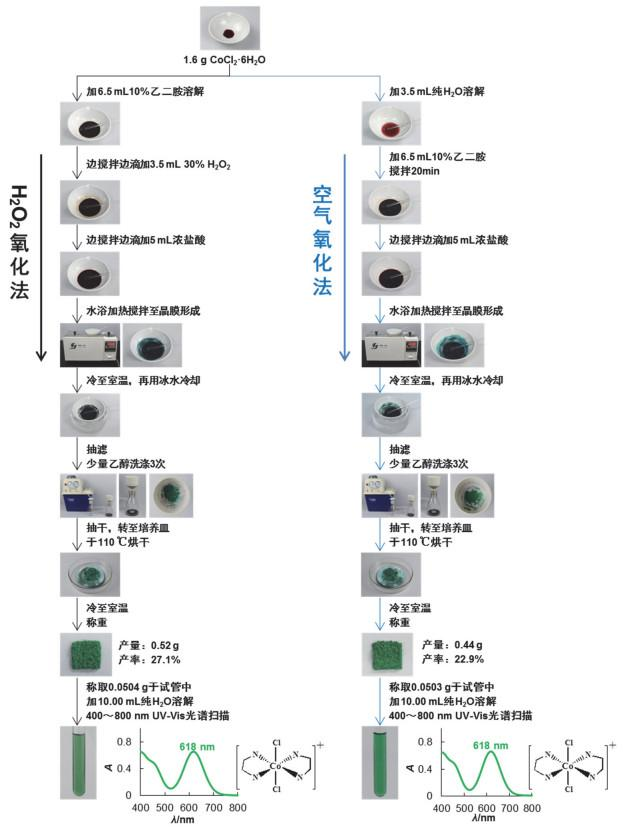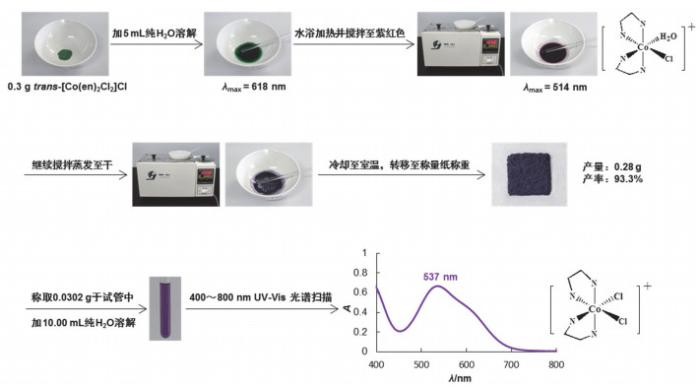## Laboratory Teaching Results and Discussions of "Preparation and Spectral Identification of trans/cis-[Co(en)2Cl2]Cl": One of the Typical Examples for Cultivating Students' Critical Thinking

Ouyang Xiaoqing, Zhang Chunyan, Pan Rui, Ruan Chanzi, Lü Yinyun, Weng Yuhua, Dong Zhiqiang, Peng Shunü, Li Huamin, Xu Zhenling, Ren Yanping,

 基金资助: 2017年福建省本科高校教育教学改革研究项目.  FBJG201702952017年度厦门大学教学改革研究项目.  JG20170204国家基础科学人才培养基金项目.  J1310024Abstract

This article mainly introduces the laboratory teaching results and discussions of "preparation and spectral identification of trans/cis-[Co(en)2Cl2]Cl", which is specially designed for the top talented chemistry-majored students in Xiamen University. Focusing on the two synthetic targets of "quality" and "quantity", i.e., how to get "pure" and "multiple" trans/cis-[Co(en)2Cl2]Cl complexes, the synthesis process and conditions were analyzed, reflected, and judged by questions and discussions based on the detailed and intuitive preparation process and UV-Visible absorption spectrum. Through this practice, we want to show how to effectively cultivate students' critical thinking in the laboratory teaching process.

Keywords： trans/cis-[Co(en)2Cl2]Cl ; Preparation and identification ; Analysis and discussion of experimental results ; Flipped Class ; Critical thinking

Ouyang Xiaoqing. Laboratory Teaching Results and Discussions of "Preparation and Spectral Identification of trans/cis-[Co(en)2Cl2]Cl": One of the Typical Examples for Cultivating Students' Critical Thinking. University Chemistry[J], 2021, 36(3): 2006069-0 doi:10.3866/PKU.DXHX202006069

### 图1### 1.1 制备原理是否可行？

$\left[\mathrm{Co}\left(\mathrm{H}_{2} \mathrm{O}\right)_{6}\right]^{2+}+2 \mathrm{en} \longrightarrow\left[\mathrm{Co}(\mathrm{en})_{2}\left(\mathrm{H}_{2} \mathrm{O}\right)_{2}\right]^{2+}+4 \mathrm{H}_{2} \mathrm{O}$

$2\left[\mathrm{Co}(\mathrm{en})_{2}\left(\mathrm{H}_{2} \mathrm{O}\right)_{2}\right]^{2+}+\mathrm{H}_{2} \mathrm{O}_{2} \longrightarrow 2\left[\mathrm{Co}(\mathrm{en})_{2}\left(\mathrm{H}_{2} \mathrm{O}\right)_{2}\right]^{3+}+2 \mathrm{OH}^{-}$

$4\left[\mathrm{Co}(\mathrm{en})_{2}\left(\mathrm{H}_{2} \mathrm{O}\right)_{2}\right]^{2+}+\mathrm{O}_{2}+2 \mathrm{H}_{2} \mathrm{O} \longrightarrow 4\left[\mathrm{Co}(\mathrm{en})_{2}\left(\mathrm{H}_{2} \mathrm{O}\right)_{2}\right]^{3+}+4 \mathrm{OH}^{-}$

$\left[\mathrm{Co}(\mathrm{en})_{2}\left(\mathrm{H}_{2} \mathrm{O}\right)_{2}\right]^{3+}+2 \mathrm{Cl}^{-} \xrightarrow[\Delta ]{过量浓盐酸} {trans}-\left[\mathrm{Co}(\mathrm{en}){ }_{2} \mathrm{Cl}_{2}\right]^{+}+2 \mathrm{H}_{2} \mathrm{O}$

### 图2## 2 如何得到又“好”又“多”的cis-[Co(en)2Cl2]Cl配合物？

${trans}-\left[\mathrm{Co}(\mathrm{en})_{2} \mathrm{Cl}_{2}\right]^{+}+\mathrm{H}_{2} \mathrm{O} \stackrel{\Delta}{\longrightarrow} {cis}-\left[\mathrm{Co}(\mathrm{en})_{2} \mathrm{Cl}\left(\mathrm{H}_{2} \mathrm{O}\right)\right]^{2+}+\mathrm{Cl}^{-}$

$c i s-\left[\mathrm{Co}(\mathrm{en})_{2} \mathrm{Cl}\left(\mathrm{H}_{2} \mathrm{O}\right)\right]^{2+}+2 \mathrm{Cl}^{-} \xrightarrow[ 蒸干]{\Delta} { cis-[ } \left.\mathrm{Co}(\mathrm{en})_{2} \mathrm{Cl}_{2}\right] \mathrm{Cl}\;(\mathrm{s})$

### 图3### 图4## 3 如何确认上述所合成的产物是顺-反异构体？

### 图5### 图6### 图7异构体 σ/cm-1 NH2不对称变形振动 N－H弯曲振动 CH2摇摆振动 Co－N或Co－Cl伸缩振动 trans-[Co(en)2Cl2]Cl 1593.30 1114.87 887.76 587.55 473.14 cis-[Co(en)2Cl2]Cl 1591.84 1563.27 1115.29 1132.65 902.04 887.76 875.51 589.80 579.59 473.47 459.18

## 参考文献 原文顺序 文献年度倒序 文中引用次数倒序 被引期刊影响因子

Preparatory Problems 43rd International Chemistry Olympiad 2011 Ankara, Turkey (第43届国际奥林匹克化学竞赛实验预备题, 土耳其, 2011年), Problem 31. [2020-06-23]. https://wenku.baidu.com/view/ed204423bcd126fff7050bb5.html

Jacewicz D. ; Pranczk J. ; Wyrzykowski D. ; Zamojc K. ; Chmurzyn'ski L. Reac. Kinet. Mech. Cat. 2014, 113, 321.

Rigg J. M. ; Sherwin E. J. lnorg. Nucl. Chem. 1965, 27, 653.

Hughes M. N. ; McWmNNIE W. R. J. Inorg. Nucl. Chem. 1966, 28, 1659.

/

 〈〉